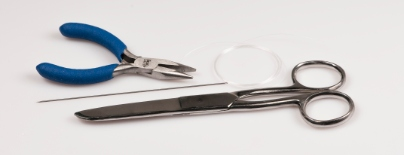# Cock-a-doodle-doo

Difficulty: 3

### Helena Chmelíková

She is able to switch from luxurious and extravagant fashion show pieces to the preparation of hobby projects,

## Material and Tools

### Tools

0.20 mm nylon line; a thin needle; scissors; flat nose pliers (toé flatten the end of the line)## Procedure

Use the peyote stitch to first create the head and then the body. Then connect both parts with the neck, decorate the chicken's body and create the stand.

### Step 1

Row 1: String and tie off 6x R8a.

Row 2: Sew 1x R8a above each gap between the R8a from the first row. A total of 6x.

Row 3: Sew 1x R8a into each gap between the R8a. A total of 6x.

Row 4: Alternately string 1x R8a and 2x R8a into the gaps. A total of 9x.

Rows 5 and 6: Sew 1x R8a into each gap between the R8a. A total of 9x.

Row 7: Sew 1x P into three consecutive gaps and sew 1x R8a into the other gaps. Then sew 2x S into one gap. Sew 1x R8a into the remaining gaps. The base for the comb and the beak.

Rows 8- 10: Sew 1x R8a into the gaps. A total of 9x. Insert a 11 - 12 mm bead into the created cup.

Row 11: String 1x R8a into two consecutive gaps and merely pass through the third one. A total of 6x. The number of gaps is reduced to 6.

Rows 12 and 13: String 1x R8a into each gap. A total of 6x.

Add 1x R8a to the middle of the circle from row 13. Emerge with the needle from one R8a in the circle. Add 1x R8a. Thread the needle into the opposite R8a in the circle. Thread the needle through the added R8a one more time. Insert the needle into the initial R8a from the second side.

1 / a

Sew the eye R8b between rows 11 and 10 between the comb and the beak. Zizag between the R8a to the second eye. Locate it between rows 3 and 4.

Zigzag to the circle from first row and add 1x R8a to the center.

/ a

## The body

Row 1: String and tie off 6x R8a.

Row 2: Add 1x R8a above every gap between the R8a from the first row. A total of 6x.

Row 3: Alternately string 1x R8a and 2x R8a into each gap. A total of 9x.

Row 4: Add 1x R8a to each gap between the R8. A total of 9x.

Row 5: String 1x R8a into two consecutive gaps and 2x R8a into the third. A total of 12x.

Row 6: String 1x R8a into three consecutive gaps and 2x R8a into the fourth. A total of 15x.

Rows 7 - 17: String 1x R8a into each gap. A total of 15x. Insert a 20 mm bead into the created cup.

Row 18: String 1x R8a into three consecutive gaps and merely pass through the fourth. A total of 12x. The number of gaps is reduced to 12.

Row 19: String 1x R8a into two consecutive gaps and merely pass through the third one. A total of 9x. The number of gaps is reduced to 9.

Row 20: String 1x R8a into each gap. A total of 9x.

Row 21. String 1x R8a into one gap and pass through the next. Alternate this procedure. A total of 6x. The number of gaps is reduced to 6.

Row 22: String 1x R8a into each gaps. A total of 6x.

Add 1x R8a to the center of the circles from rows 1 and 23.

2 / a

3 / a

## The wings

Use 1x R8a in rows 3 and 4 on the front part of the body and in rows 20 and 19 on the opposite side. Use one R8a in row 6 or 17 on the back. Thread the line through an R8a on the chest from the lower circle in the neck in about the 4th line. String 15x R10. Thread the line through the R8a on the back. Once again thread the line through the fifteenth R10. Add 14x R10. Tie off the line and sew it in.

4 / a

## The tail

Count off 8 lines from the lower circle on the neck. Thread the line from the bottom of the R8a in row 10. String 15x R10. Thread the line through the R8a in row 12 from above. Insert the line into the R8a in row 14 from below. String 15x R10. Thread the line through the R8a in row 16 from above. Zigzag through the R8a to the 9th line from the neck. Use the R8a v rows 17, 15, 13 and 11. Zigzag through the R8a to the 10th line from the neck. Use rows 8, 10, 12 a 14.

5 / a

## The stand

Alternately string triangles (Tr) and R10: 13 of each. Thread the line through them once again. You can use 20x R6 as a replacement. Then thread the line through them once again. The chicken is now finished.

6 / a